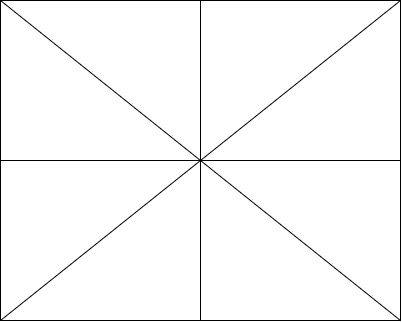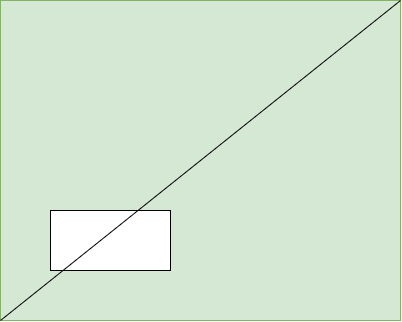# Puzzle | Splitting a Cake with a Missing Piece in two equal portion

• Difficulty Level : Easy
• Last Updated : 09 Aug, 2021

Problem Statement: There are three friends who ordered a rectangular cake. One of them was in a hurry so he cut a rectangular piece from the cake. The piece could be of any arbitrary size(less than the size of the cake) and rotation. Now the remaining two friends want to divide the remaining cake into two equal portions with a single straight cut.

Note:

• The single straight cut could pass through the cut-out portion.
• They both need an equal amount of cream and toppings. So they can not cut along the height of the cake.

Observation:• All lines in the above diagram divide the rectangle into two equal halves.
• The observation is that any line that passes through the center of the rectangle divides the rectangle into two equal halves.

Solution:

• By extending our observation and create a line such that it passes through both the center of the cake as well as the center of the missing piece.
• Such a line would divide the cake into two equal halves.My Personal Notes arrow_drop_up